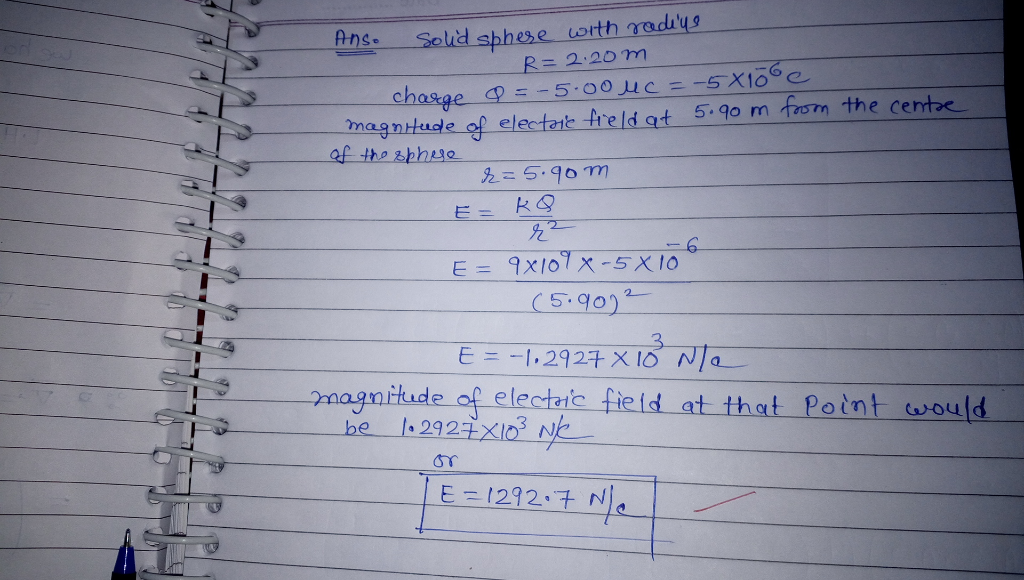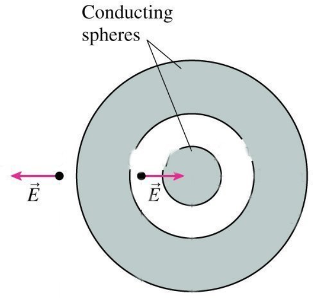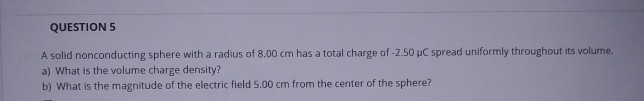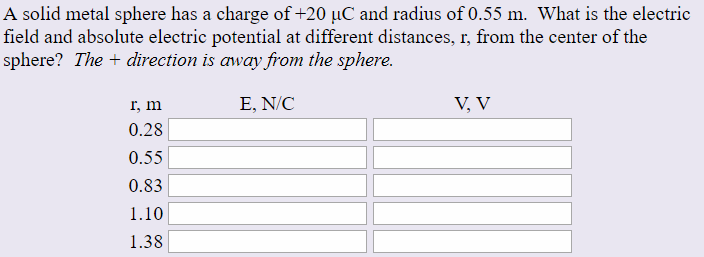Question

A solid metal sphere with a radius of 2.20 m has a total load of - 5.00 µC. What is the magnitude of the electric field at a distance of 5.90 m from the center of the sphere?#### Earn Coins

Coins can be redeemed for fabulous gifts.

Similar Homework Help Questions
• ### A solid metal sphere of radius 3.00 m carries a total charge of -7.00 μC .

A solid metal sphere of radius 3.00 m carries a total charge of -7.00 μC . Part A What is the magnitude of the electric field at a distance from the sphere's center of 0.200 m? Part B What is the magnitude of the electric field at a distance from the sphere's center of 2.95 m? Part C What is the magnitude of the electric field at a distance from the sphere's center of 3.05 m? Part D What is the magnitude of the electric field at a...

• ### Given: A solid metal sphere of radius 2.00 m carries a total charge of -5.30 μC...

Given: A solid metal sphere of radius 2.00 m carries a total charge of -5.30 μC . A. What is the magnitude of the electric field at a distance from the sphere's center of 0.10 m? B. What is the magnitude of the electric field at a distance from the sphere's center of 1.95 m?

• ### The figure below shows a solid metal sphere at the centre of a hollow metal sphere. The radius of the solid metal sphere Is 4.53cm, the inner radius of the hollow sphere is 9.2cmThe figure below shows a solid metal sphere at the centre of a hollow metal sphere. The radius of the solid metal sphere Is 4.53cm, the inner radius of the hollow sphere is 9.2cm, and the outer radlus of the hollow sphere is 13.2cm. The electric field shown on the diagram inside the hollow sphere, at a distance of 7.83cm from the centre of the solid metal sphere, has magnitude of 14570N/C. The strength of the electric field at a...

• ### A solid sphere of radius 40.0 cm has a total positive charge of 26.5 µC uniformly...

A solid sphere of radius 40.0 cm has a total positive charge of 26.5 µC uniformly distributed throughout its volume. Calculate the magnitude of the electric field at the following distances. (c) 40.0 cm from the center of the sphere in kN/C (d) 72.0 cm from the center of the sphere in kN/C Thanks

• ### A solid conducting sphere of radius 2.00 cm has a charge 11.00 µC. A conducting spherical...

A solid conducting sphere of radius 2.00 cm has a charge 11.00 µC. A conducting spherical shell of inner radius 4.00 cm and outer radius 5.00 cm is concentric with the solid sphere and has a total charge of -1.00 µC. (Take radially outward as the positive direction.) (a) Find the electric field at r = 1.00 cm from the center of this charge configuration. MN/C (b) Find the electric field at r = 3.00 cm from the center of...

• ### QUESTION 5 A solid nonconducting sphere with a radius of 8.00 cm has a total charge...QUESTION 5 A solid nonconducting sphere with a radius of 8.00 cm has a total charge of -2.50 C spread uniformly throughout its volume. a) What is the volume charge density? b) What is the magnitude of the electric field 5.00 cm from the center of the sphere?

• ### A solid sphere of radius 40.0 cm has a total positive charge of 34.4 µC uniformly...

A solid sphere of radius 40.0 cm has a total positive charge of 34.4 µC uniformly distributed throughout its volume. Calculate the magnitude of the electric field at the following distances. (a) 0 cm from the center of the sphere _____kN/C (b) 10.0 cm from the center of the sphere ______kN/C (c) 40.0 cm from the center of the sphere _____kN/C (d) 74.0 cm from the center of the sphere _______kN/C

• ### A nonconducting solid sphere of radius 2.20 cm carries a uniformly distributed positive charge of 7.00×10-9...

A nonconducting solid sphere of radius 2.20 cm carries a uniformly distributed positive charge of 7.00×10-9 C. Calculate the magnitude of the electric field at a point 1.40 cm away from the center of the sphere. Calculate the magnitude of the electric field at a point 3.20 cm away from the center of the sphere.

• ### A solid metal sphere has a charge of +20 μC and radius of 0.55 m. What...A solid metal sphere has a charge of +20 μC and radius of 0.55 m. What is the electric field and absolute electric potential at different distances, r, from the center of the sphero he irectois away from the sphare. E, N/C r, m 0.28 0.55 0.83 1.10 1.38

• ### Charge of uniform volume density ρ = 4.30 µC/m3 fills a nonconducting solid sphere of radius...

Charge of uniform volume density ρ = 4.30 µC/m3 fills a nonconducting solid sphere of radius 3.50 cm. What is the magnitude of the electric field (a) 2.20 cm and (b) 4.90 cm from the sphere's center?